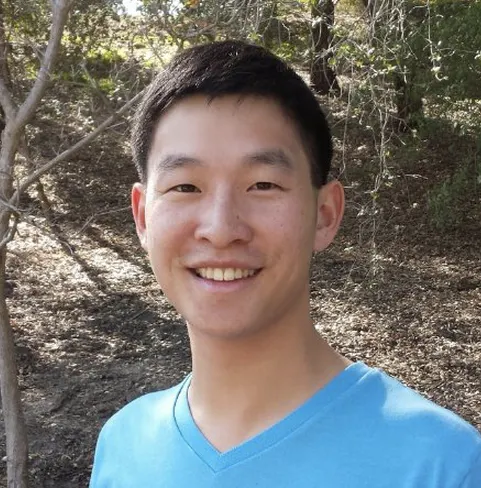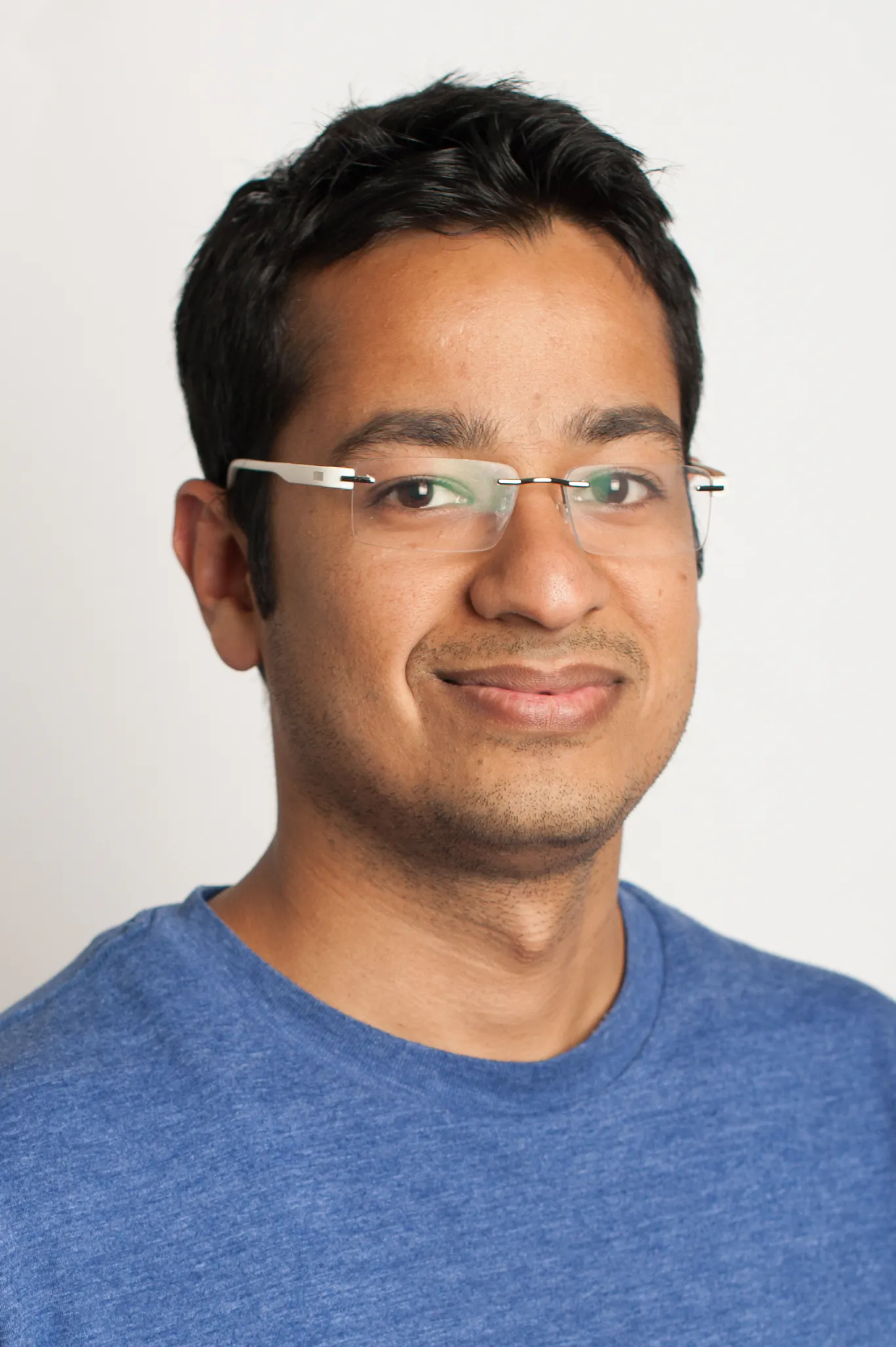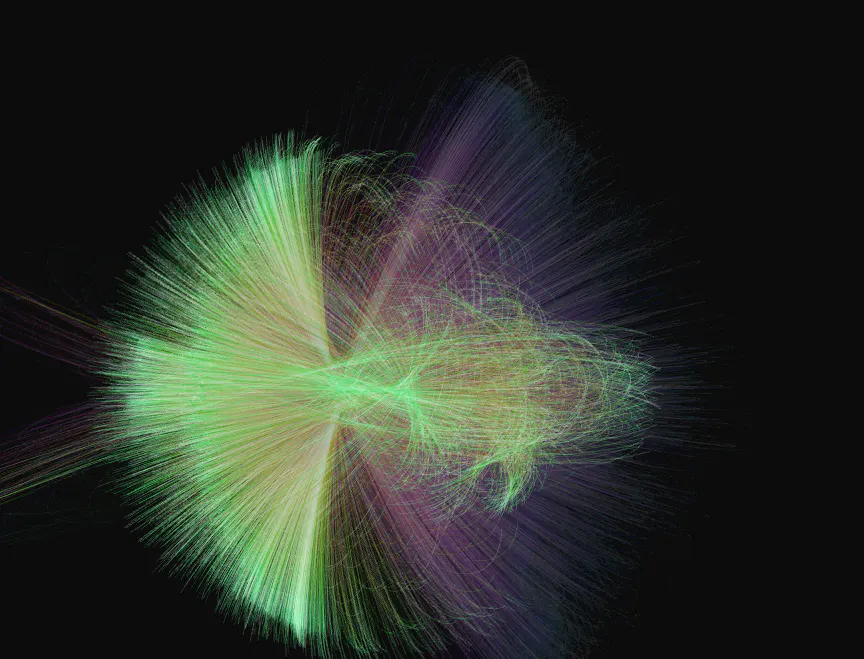Published

# Paper Instruments

Make paper instruments!

Work in progress9,409## Things used in this project

### Hardware componentsArduino UNO & Genuino UNO
×1

## Code

### MakerFaireProcessingFinal.pde

Java
MakerFaireProcessingFinal.pde
```/* OpenProcessing Tweak of *@*http://www.openprocessing.org/sketch/2363*@* */
/* !do not delete the line above, required for linking your tweak if you upload again */

import processing.serial.*;
import java.util.ArrayList;
import themidibus.*;

String val;

int maxNumberOfSensors = 12;       // Arduino has 6 analog inputs, so I chose 6

boolean fontInitialized = false;  // whether the font's been initialized
PFont myFont;                     // font for writing text to the window
int STARTED =0;

Serial myPort;
particle[] Z = new particle;
float colour = random(1);

boolean PRESSED=false;
int screenCounter=0;
PVector v1;
long counter=0;
MidiBus myMidi;
ArrayList<Note> keyboard;
boolean[] keyIsPressed;
int[] lastPressed;
final float KEYDOWN_THRESHOLD = 1000f;

void setup() {
smooth();
size(1800, 1000, P2D);
background(255);
v1=new PVector(width/2, height/2);
for (int i = 0; i < Z.length; i++) {
Z[i] = new particle( random(width), random(height), 0, 0, 1 );
}

/* Setup all sound */
myMidi = new MidiBus(this, -1, 0);
myMidi.sendTimestamps(false);
keyboard = new ArrayList<Note>();
int channel = 0;
int velocity = 127;
for(int pitch = 71; pitch > 59; pitch--) {
}

keyIsPressed = new boolean[keyboard.size()];
lastPressed = new int[keyboard.size()];

frameRate(60);
colorMode(RGB, 255);

String portName = Serial.list();
myPort = new Serial(this, portName, 115200);
myPort.clear();
myPort.bufferUntil('\n');  // don't generate a serialEvent() until you get a newline (\n) byte
}

void draw() {
filter(INVERT);
float r;
stroke(0);
fill(255, 10);

rect(0, 0, width, height);

colorMode(HSB, 1);
for (int i = 0; i < Z.length; i++) {
if (PRESSED==true) {
Z[i].gravitate (new particle(v1.x, v1.y, 0, 0, 5));
//fill(colour, 10);
//ellipse(v1.x, v1.y, 20, 20);
}
else {
//Z[i].gravitate (new particle(width/2, height/2, 0, 0, 1));
screenCounter++;
if(screenCounter<5000){
// Z[i].repel (new particle(v1.x, v1.y, 0, 0, 1));
screenCounter=0;
}
Z[i].deteriorate();
}
Z[i].update();
r = float(i)/Z.length;
stroke( colour, pow(r, 0.1), 1-r, 0.15 );
Z[i].display();
}
colorMode(RGB, 255);

//colour+=random(0.01);
if ( colour > 1 ) {
colour = colour%1;
}

filter(INVERT);
}

void startNote(int keyIndex) {
if(!keyIsPressed[keyIndex]) {
Note note = keyboard.get(keyIndex);
myMidi.sendNoteOn(note);
keyIsPressed[keyIndex] = true;
}
}

void delay(int time) {
int current = millis();
while(millis() < current + time) {
}
}

void endNote(int keyIndex) {
if(keyIsPressed[keyIndex]) {
Note note = keyboard.get(keyIndex);
myMidi.sendNoteOff(note);
keyIsPressed[keyIndex] = false;
}
}

void serialEvent (Serial myPort) {
String inString = myPort.readStringUntil('\n');  // get the ASCII string
if (inString != null) {  // if it's not empty
inString = trim(inString);  // trim off any whitespace
/*
System.out.format("Length: %d\n", length);
double[] incomingValues = new double[length];
for(int i = 0; i < length; i++) {
incomingValues[i] = Double.valueOf(tmp[i]);
}
System.out.format("Double: %f\n", incomingValues);
*/
String[] tmp = split(inString, ",");
float[] incomingValues = float(tmp);
int length = incomingValues.length;
if (length == maxNumberOfSensors+1 && length > 0) {
for (int i = 0; i < length-1; i++) {
counter+=incomingValues[i];
/* Key is Pressed */
if (incomingValues[i] > KEYDOWN_THRESHOLD) {
PRESSED=true;
v1.x=width/2-(i-6)*(width/2)/8 ;
v1.y=height/2;
colour+=random(0.01);
if (i==1|i==3|i==5|i==8|i==10) {
v1.y=height/2+random(-height/2, height/2);
}
startNote(i);
}

/* Key is not pressed */
if (counter<1000) {
PRESSED=false;
endNote(i);
}
if (i==11) {
counter=0;
}

/*if (i>6) {
v1.x=random(width/4, width/2);
}
else {
v1.x=(width/2);
}
*/
}
}
}
}
```

### PianoGuitarHackster.ino

Plain text
PianoGuitarHackster.ino
```#include <CapacitiveSensor.h>
float matrixValues;

byte controlPins[] = {
B00000000,
B10000000,
B01000000,
B11000000,
B00100000,
B10100000,
B01100000,
B11100000,
B00010000,
B10010000,
B01010000,
B11010000,
B00110000,
B10110000,
B01110000,
B11110000 };

CapacitiveSensor   cs_8_0 = CapacitiveSensor(8,A0);
void setup()
{
Serial.begin(115200);
cs_8_0.set_CS_AutocaL_Millis(0xFFFFFFFF);
DDRD = B11111111; // set PORTD (digital 7~0) to outputs
}

void setPin(int outputPin)
// function to select pin on 74HC4067
{
PORTD = controlPins[outputPin];
}

void loop()
{
for (int i = 0; i < 12; i++)
{
setPin(i); // choose an input pin on the 74HC4067
float total1 =  cs_8_0.capacitiveSensor(30);
matrixValues[i]=total1;
Serial.print(matrixValues[i]);
Serial.print(",");
}
Serial.println();
}
```

## Credits

### Karen Yee

2 projects • 5 followers
tech enthusiast, product management, finance & analytics, cognitive science

### Alex Yee

2 projects • 2 followers
hardware hacker, BLE enthusiast, Arduino hackster

### Daniel Chen

2 projects • 1 follower

### Anupam Jindal

2 projects • 4 followers
Technology lover, iOS developer, Product Manager, Java developer

### Manny Karyampudi

2 projects • 4 followers
Generalist Engineer | Android Development | Web Development | Business Analysis